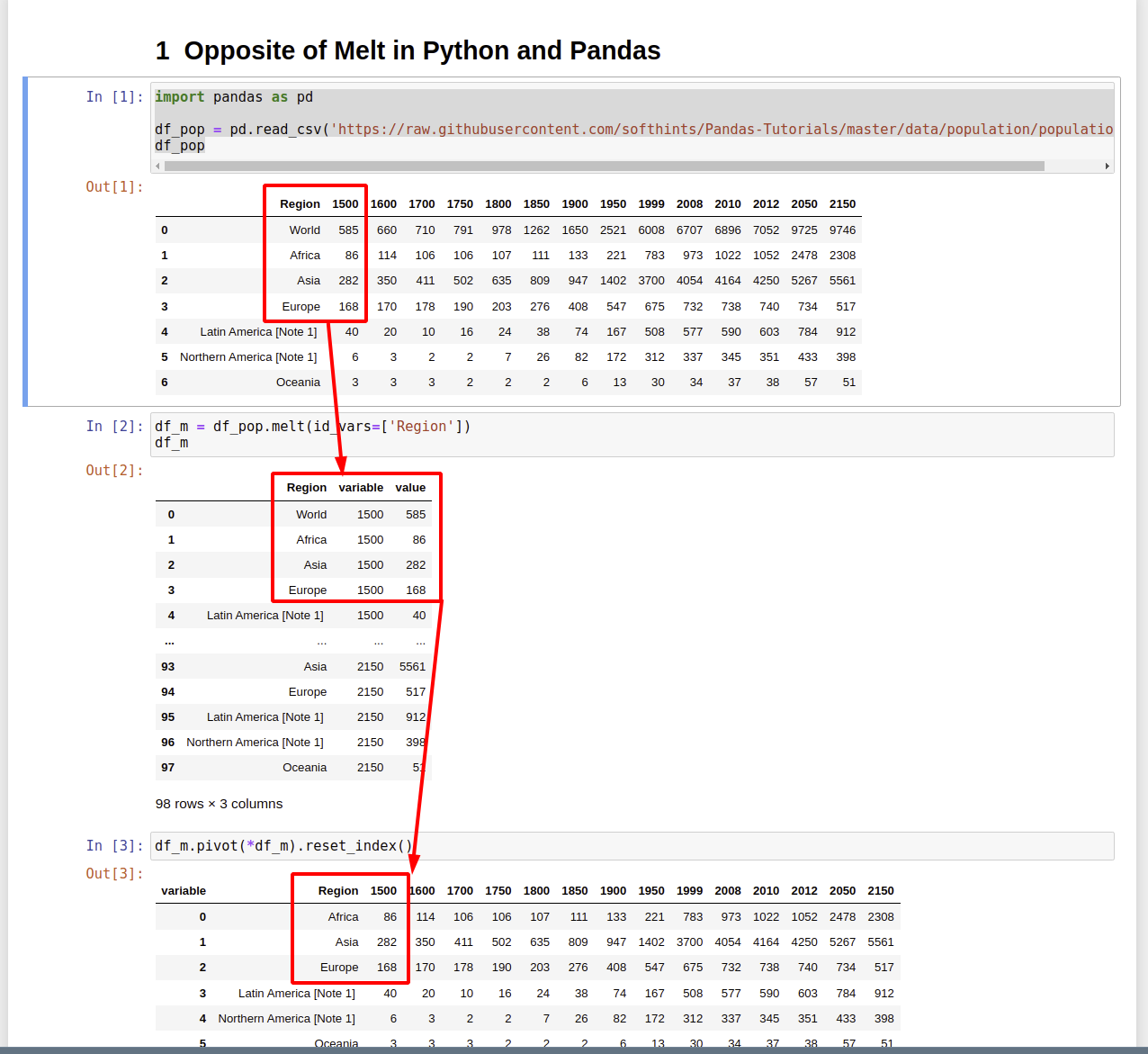In this short guide, you'll see what is the opposite operation of melt in Pandas and Python. You can find a useful example.

The short answer of the question above is:

``````df_m.pivot(*df_m).reset_index()
``````

Let's show a detailed example on the above:

``````import pandas as pd

df_pop
``````
Region 1500 1600 2010 2012 2050 2150
World 585 660 6896 7052 9725 9746
Africa 86 114 1022 1052 2478 2308
Asia 282 350 4164 4250 5267 5561
Europe 168 170 738 740 734 517
Latin America [Note 1] 40 20 590 603 784 912

In order to demo the opposite of melt operation let's perform melt on the above data:

``````df_m = df_pop.melt(id_vars=['Region'])
df_m
``````

The DataFrame `df_m` has the next data inside:

Region variable value
World 1500 585
Africa 1500 86
Asia 1500 282
Europe 1500 168
Latin America [Note 1] 1500 40
Northern America [Note 1] 1500 6
Oceania 1500 3
World 1600 660
Africa 1600 114
Asia 1600 350

So the years which were stored as columns after the melt operation are transformed to rows.

``````Index(['Region', '1500', '1600', '1700', '1750', '1800', '1850', '1900',
'1950', '1999', '2008', '2010', '2012', '2050', '2150'],
dtype='object')
``````

after melt operation we end with 3 columns:

• Region - the column on which we do the melt operation
• variable - which is the column name of the old DataFrame
• value - the corresponding value of the first DataFrame

## Reverse Melt Operation in Python and Pandas

Now let's reverse the melt which was performed above. We are going to work with DataFrame df_m.

There are several ways of reversing melt operation in Pandas. In this post we will demonstrate the one which uses method `pivot` and `reset_index`:

``````df_m.pivot(*df_m).reset_index()
``````

If you like to get the original DataFrame from you will need to rename the columns by `.rename_axis(None, axis='columns')`. So the full code will become:

``````df_m.pivot(*df_m).reset_index().rename_axis(None, axis='columns')
``````

The difference between those two is the name of the index. Without `.rename_axis(None, axis='columns')` we will get:

Index(['Region', '1500', '1600', '1700', '1750', '1800', '1850', '1900',
'1950', '1999', '2008', '2010', '2012', '2050', '2150'],
dtype='object', name='variable')

with `.rename_axis(None, axis='columns')` we will have different index name after the pivot.:

Index(['Region', '1500', '1600', '1700', '1750', '1800', '1850', '1900',
'1950', '1999', '2008', '2010', '2012', '2050', '2150'],
dtype='object')Note: Please note that the index is sorted alphabetically and doesn't match the original sort.

## Opposite of melt on few values only

Finally let's see the example when you like to melt or reverse it on a few variables. This can be done by using parameter `value_vars` of method `melt`:

``````df_m = df_pop.melt(id_vars=['Region'], value_vars=['1500', '1600', '1700'])
df_m
``````

result:

Region variable value
World 1500 585
Africa 1500 86
Asia 1500 282
Europe 1500 168
Latin America [Note 1] ​ 1500 40

The reverse operation have additional parameter `column`:

``````df_m.pivot(index='Region', columns='variable')['value']
``````
variable 1500 1600 1700
Region
Africa 86 114 106
Asia 282 350 411
Europe 168 170 178
Latin America [Note 1] 40 20 10
Northern America [Note 1] 6 3 2